Documentation

### This is machine translation

Mouseover text to see original. Click the button below to return to the English version of the page.

Note: This page has been translated by MathWorks. Click here to see
To view all translated materials including this page, select Country from the country navigator on the bottom of this page.

# sbiopredictionci

Compute confidence intervals for model predictions (requires Statistics and Machine Learning Toolbox)

## Syntax

``ci = sbiopredictionci(fitResults)``
``ci = sbiopredictionci(fitResults,Name,Value)``

## Description

example

````ci = sbiopredictionci(fitResults)` computes 95% confidence intervals for the model simulation results from `fitResults`, an `NLINResults object` or `OptimResults object` returned by `sbiofit`. `ci` is a `PredictionConfidenceInterval` object that contains the computed confidence interval data.```
````ci = sbiopredictionci(fitResults,Name,Value)` uses additional options specified by one or more `Name,Value` pair arguments.```

## Examples

collapse all

Load Data

Load the sample data to fit. The data is stored as a table with variables ID , Time , CentralConc , and PeripheralConc. This synthetic data represents the time course of plasma concentrations measured at eight different time points for both central and peripheral compartments after an infusion dose for three individuals.

```clear all load data10_32R.mat gData = groupedData(data); gData.Properties.VariableUnits = {'','hour','milligram/liter','milligram/liter'}; sbiotrellis(gData,'ID','Time',{'CentralConc','PeripheralConc'},'Marker','+',... 'LineStyle','none'); ```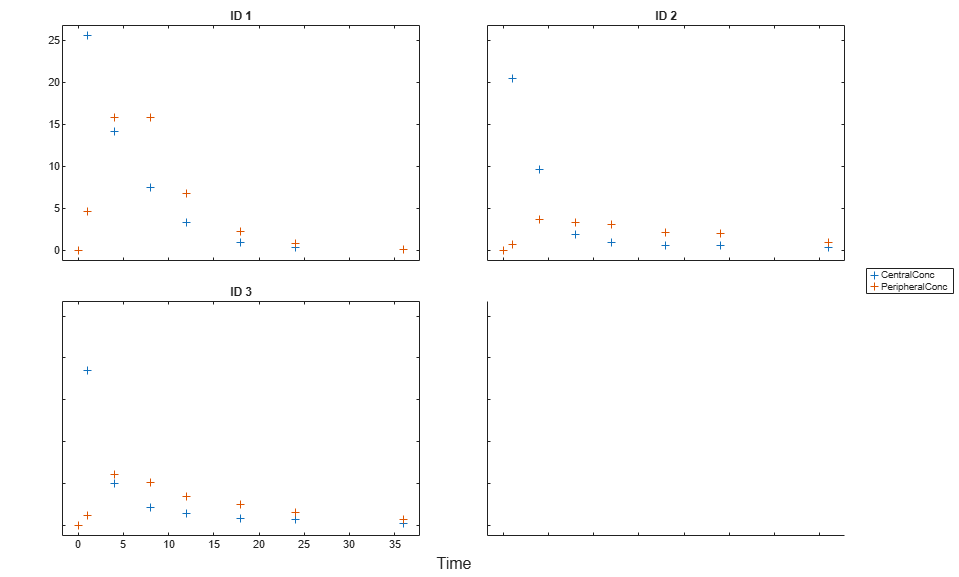Create Model

Create a two-compartment model.

```pkmd = PKModelDesign; pkc1 = addCompartment(pkmd,'Central'); pkc1.DosingType = 'Infusion'; pkc1.EliminationType = 'linear-clearance'; pkc1.HasResponseVariable = true; pkc2 = addCompartment(pkmd,'Peripheral'); model = construct(pkmd); configset = getconfigset(model); configset.CompileOptions.UnitConversion = true; ```

Define Dosing

Define the infusion dose.

```dose = sbiodose('dose','TargetName','Drug_Central'); dose.StartTime = 0; dose.Amount = 100; dose.Rate = 50; dose.AmountUnits = 'milligram'; dose.TimeUnits = 'hour'; dose.RateUnits = 'milligram/hour'; ```

Define Parameters

Define the parameters to estimate. Set the parameter bounds for each parameter. In addition to these explicit bounds, the parameter transformations (such as log, logit, or probit) impose implicit bounds.

```responseMap = {'Drug_Central = CentralConc','Drug_Peripheral = PeripheralConc'}; paramsToEstimate = {'log(Central)','log(Peripheral)','Q12','Cl_Central'}; estimatedParam = estimatedInfo(paramsToEstimate,... 'InitialValue',[1 1 1 1],... 'Bounds',[0.1 3;0.1 10;0 10;0.1 2]); ```

Fit Model

Perform an unpooled fit, that is, one set of estimated parameters for each patient.

```unpooledFit = sbiofit(model,gData,responseMap,estimatedParam,dose,'Pooled',false); ```

Perform a pooled fit, that is, one set of estimated parameters for all patients.

```pooledFit = sbiofit(model,gData,responseMap,estimatedParam,dose,'Pooled',true); ```

Compute Confidence Intervals for Estimated Parameters

Compute 95% confidence intervals for each estimated parameter in the unpooled fit.

```ciParamUnpooled = sbioparameterci(unpooledFit); ```

Display Results

Display the confidence intervals in a table format. For details about the meaning of each estimation status, see Parameter Confidence Interval Estimation Status.

```ci2table(ciParamUnpooled) ```
```ans = 12x7 table Group Name Estimate ConfidenceInterval Type Alpha Status _____ ____________ ________ __________________ ________ _____ ___________ 1 'Central' 1.422 1.1533 1.6906 Gaussian 0.05 estimable 1 'Peripheral' 1.5629 0.83143 2.3551 Gaussian 0.05 constrained 1 'Q12' 0.47159 0.20093 0.80247 Gaussian 0.05 constrained 1 'Cl_Central' 0.52898 0.44842 0.60955 Gaussian 0.05 estimable 2 'Central' 1.8322 1.7893 1.8751 Gaussian 0.05 success 2 'Peripheral' 5.3368 3.9133 6.7602 Gaussian 0.05 success 2 'Q12' 0.27641 0.2093 0.34351 Gaussian 0.05 success 2 'Cl_Central' 0.86034 0.80313 0.91755 Gaussian 0.05 success 3 'Central' 1.6657 1.5818 1.7497 Gaussian 0.05 success 3 'Peripheral' 5.5632 4.7557 6.3708 Gaussian 0.05 success 3 'Q12' 0.78361 0.65581 0.91142 Gaussian 0.05 success 3 'Cl_Central' 1.0233 0.96375 1.0828 Gaussian 0.05 success ```

Plot the confidence intervals. If the estimation status of a confidence interval is `success`, it is plotted in blue (the first default color). Otherwise, it is plotted in red (the second default color), which indicates that further investigation into the fitted parameters may be required. If the confidence interval is `not estimable`, then the function plots a red line with a centered cross. If there are any transformed parameters with estimated values 0 (for the log transform) and 1 or 0 (for the probit or logit transform), then no confidence intervals are plotted for those parameter estimates. To see the color order, type `get(groot,'defaultAxesColorOrder')`.

Groups are displayed from left to right in the same order that they appear in the `GroupNames` property of the object, which is used to label the x-axis. The y-labels are the transformed parameter names.

```plot(ciParamUnpooled) ```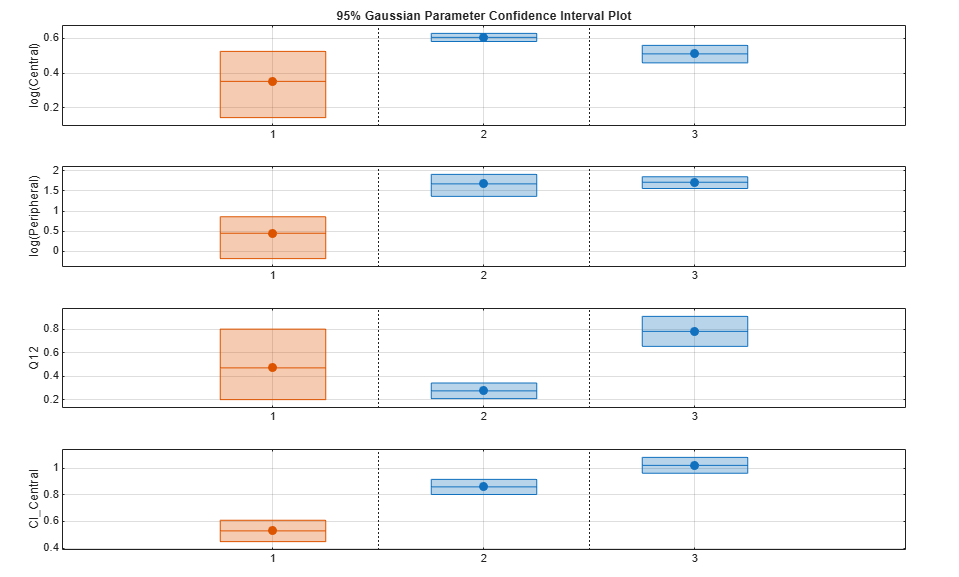Compute the confidence intervals for the pooled fit.

```ciParamPooled = sbioparameterci(pooledFit); ```

Display the confidence intervals.

```ci2table(ciParamPooled) ```
```ans = 4x7 table Group Name Estimate ConfidenceInterval Type Alpha Status ______ ____________ ________ __________________ ________ _____ ___________ pooled 'Central' 1.6626 1.3287 1.9965 Gaussian 0.05 estimable pooled 'Peripheral' 2.687 0.89848 4.8323 Gaussian 0.05 constrained pooled 'Q12' 0.44956 0.11445 0.85152 Gaussian 0.05 constrained pooled 'Cl_Central' 0.78493 0.59222 0.97764 Gaussian 0.05 estimable ```

Plot the confidence intervals. The group name is labeled as "pooled" to indicate such fit.

```plot(ciParamPooled) ```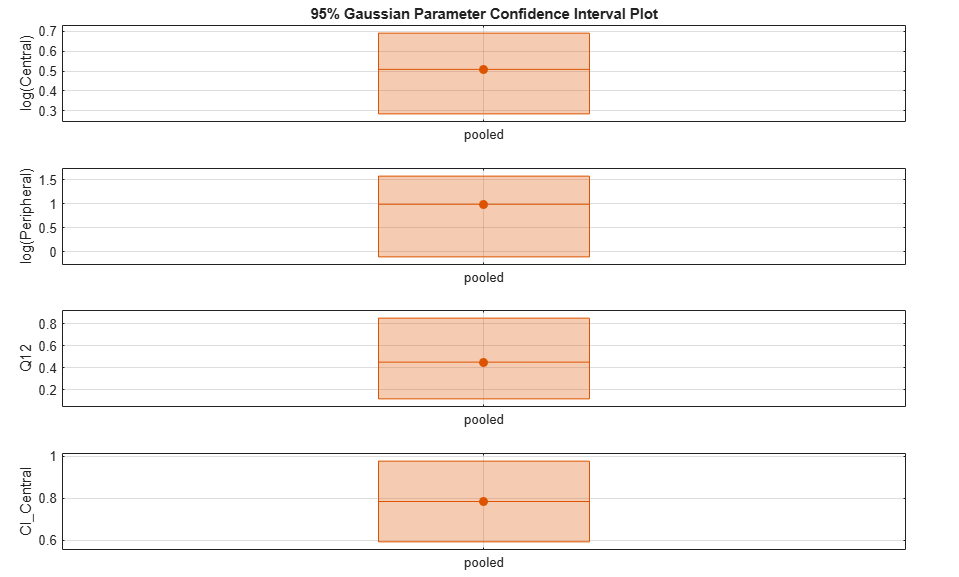Plot all the confidence interval results together. By default, the confidence interval for each parameter estimate is plotted on a separate axes. Vertical lines group confidence intervals of parameter estimates that were computed in a common fit.

```ciAll = [ciParamUnpooled;ciParamPooled]; plot(ciAll) ```You can also plot all confidence intervals in one axes grouped by parameter estimates using the 'Grouped' layout.

```plot(ciAll,'Layout','Grouped') ```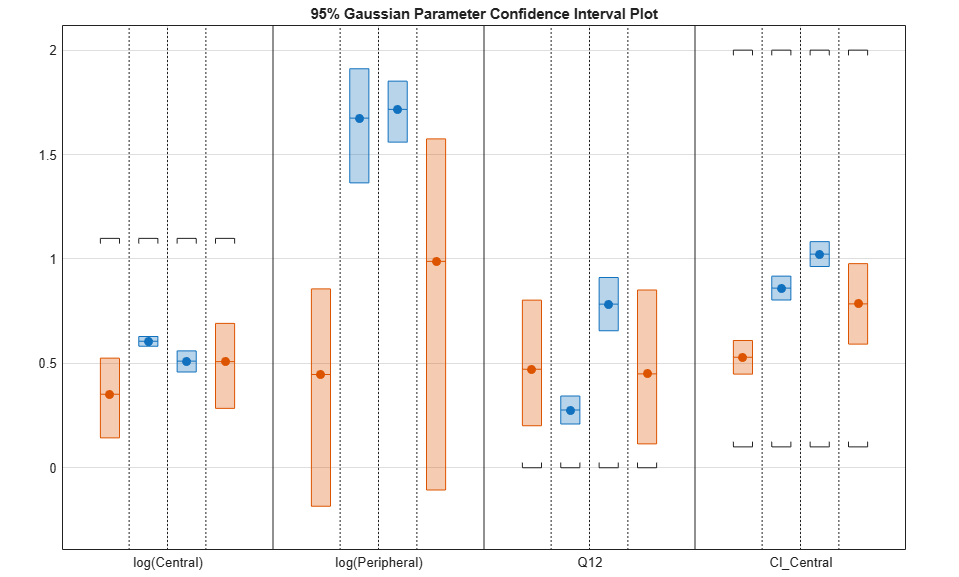In this layout, you can point to the center marker of each confidence interval to see the group name. Each estimated parameter is separated by a vertical black line. Vertical dotted lines group confidence intervals of parameter estimates that were computed in a common fit. Parameter bounds defined in the original fit are marked by square brackets. Note the different scales on the y-axis due to parameter transformations. For instance, the y-axis of `Q12` is in the linear scale, but that of `Central` is in the log scale due to its log transform.

Compute Confidence Intervals for Model Predictions

Calculate 95% confidence intervals for the model predictions, that is, simulation results using the estimated parameters.

```% For the pooled fit ciPredPooled = sbiopredictionci(pooledFit); % For the unpooled fit ciPredUnpooled = sbiopredictionci(unpooledFit); ```

Plot Confidence Intervals for Model Predictions

The confidence interval for each group is plotted in a separate column, and each response is plotted in a separate row. Confidence intervals limited by the bounds are plotted in red. Confidence intervals not limited by the bounds are plotted in blue.

```plot(ciPredPooled) ```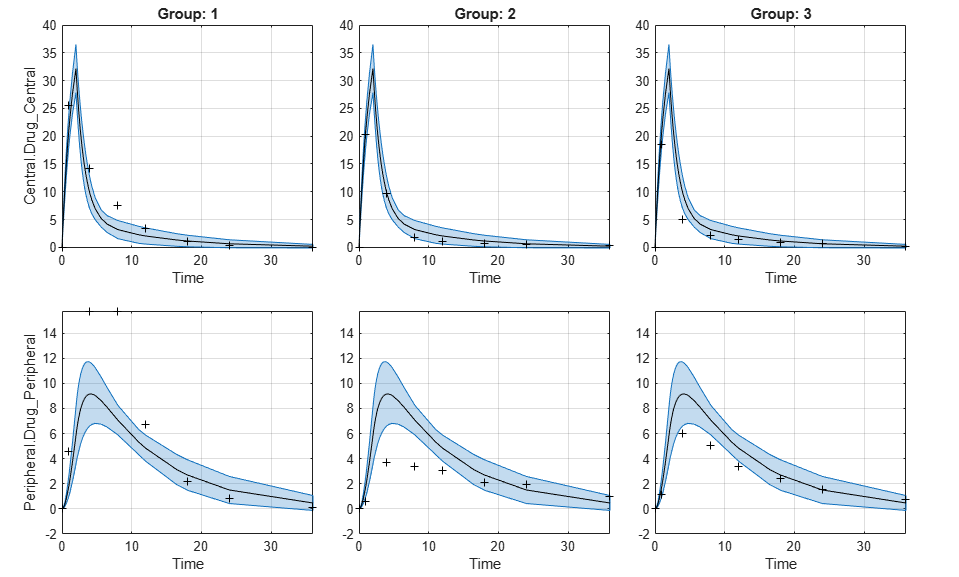```plot(ciPredUnpooled) ```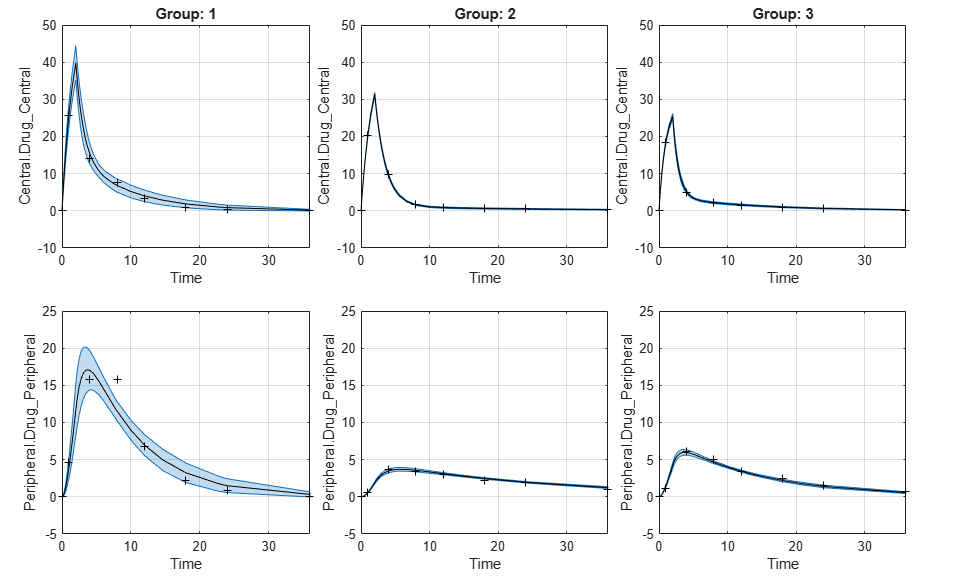## Input Arguments

collapse all

Parameter estimation results from `sbiofit`, specified as an `NLINResults object`, `OptimResults object`, or a vector of objects for unpooled fits that were returned from the same `sbiofit` call.

### Name-Value Pair Arguments

Specify optional comma-separated pairs of `Name,Value` arguments. `Name` is the argument name and `Value` is the corresponding value. `Name` must appear inside quotes. You can specify several name and value pair arguments in any order as `Name1,Value1,...,NameN,ValueN`.

Example: `'Alpha',0.01,'Type','bootstrap'` specifies to compute a 99% confidence interval using the bootstrap method.

Confidence level, `(1-Alpha) * 100%`, specified as the comma-separated pair consisting of `'Alpha'` and a positive scalar between 0 and 1. The default value is `0.05`, meaning a 95% confidence interval is computed.

Example: `'Alpha',0.01`

Confidence interval type, specified as the comma-separated pair consisting of `'Type'` and a character vector. The valid choices are:

• `'gaussian'`– Use the Gaussian approximation of the distribution of the linearized model responses around the parameter estimates.

• `'bootstrap'`– Compute confidence intervals using the bootstrap method.

Example: `'Type','bootstrap'`

Number of samples for bootstrapping, specified as the comma-separated pair consisting of `'NumSamples'` and a positive integer. This number defines the number of fits that are performed during the confidence interval computation to generate bootstrap samples. The smaller the number is, the faster the computation of the confidence intervals becomes, at the cost of decreased accuracy.

Example: `'NumSamples',500`

Level of display returned to the command line, specified as the comma-separated pair consisting of `'Display'` and a character vector. `'off'` (default) or `'none'` displays no output. `'final'` displays a message when the computation finishes.

Example: `'Display','final'`

Logical flag to compute confidence intervals in parallel, specified as the comma-separated pair consisting of `'UseParallel'` and `true` or `false`. By default, the parallel options in the original fit are used. If this argument is set to `true` and Parallel Computing Toolbox™ is available, the parallel options in the original fit are ignored, and confidence intervals are computed in parallel.

For the Gaussian confidence intervals:

• If the input `fitResults` is a vector of results objects, then the computation of confidence intervals for each object is performed in parallel. The Gaussian confidence intervals are quick to compute. So, it might be more beneficial to parallelize the original fit (`sbiofit`) and not set `UseParallel` to true for `sbiopredictionci`.

For the Bootstrap confidence intervals:

• The function forwards the `UseParallel` flag to `bootci`. There is no parallelization over the input vector of results objects.

### Note

If you have a global stream for random number generation with a number of substreams to compute in parallel in a reproducible fashion, `sbiopredictionci` first checks to see if the number of workers is same as the number of substreams. If so, the function sets `UseSubstreams` to `true` in the `statset` option and passes to `bootci`. Otherwise, the substreams are ignored by default.

Example: `'UseParallel',true`

## Output Arguments

collapse all

Confidence interval results, returned as a `PredictionConfidenceInterval` object. For an unpooled fit, `ci` can be a vector of `PredictionConfidenceInterval` objects.

## More About

collapse all

### Gaussian Confidence Interval Calculation for Model Predictions

The model is linearized around the parameter estimates Pest that are obtained from the fit results returned by `sbiofit`. The `CovarianceMatrix` is transformed using the linearized model. In addition, implicit parameter bounds (`log`, `probit`, or `logit` parameter transforms specified in the original fit) and explicit parameter bounds (if specified in the original fit) are also mapped through the linearized model.

To linearize the model, `sbiopredictionci` first checks to see if the sensitivity analysis feature is turned on in the original fit. If the feature is on, the function uses the Jacobian computed via the complex step differentiation. If the feature is off, the Jacobian is computed using finite differencing. Finite differencing can be inaccurate, and consider turning on the sensitivity analysis feature when you run `sbiofit`.

The function uses the transformed `CovarianceMatrix` and computes the Gaussian confidence intervals for each estimated model response at every time step.

In cases where the confidence interval is constrained by the parameter bounds defined in the original fit, the confidence interval bounds are adjusted according to the approach described by Wu, H. and Neale, M. .

#### Setting Estimation Status

• For each model response, the function first decides whether the confidence interval is unbounded. If so, the estimation status of the corresponding model response is set to ```not estimable```.

• Otherwise, if the confidence interval for a response is constrained by a parameter bound defined in the original fit, the function sets its status to `constrained`. Parameter transformations (such as `log`, `probit`, or `logit`) impose implicit bounds on the estimated parameters, for example, positivity constraints. Such bounds can lead to the overestimation of confidence, that is, the confidence interval can be smaller than expected.

• If no confidence interval has the estimation status ```not estimable``` or `constrained`, then the function sets the estimation statuses of all model responses to `success`. Otherwise, the estimation statuses of remaining model responses are set to `estimable`.

### Bootstrap Confidence Interval Calculation

The `bootci` function from Statistics and Machine Learning Toolbox™ is used to compute the bootstrap confidence intervals. The first input nboot is the number of samples (`NumSamples`), and the second input bootfun is a function that performs these actions.

• Resample the data (independently within each group, if multiple groups are available).

• Run a parameter fit with the resampled data.

• Simulate the model using the estimated parameters to get model responses.

• Return model responses.

#### Setting Estimation Status

The estimation status is always set to `estimable` since the function cannot determine if the confidence intervals are constrained by the bounds on the parameter estimates.

 Wu, H., and M.C. Neale. "Adjusted Confidence Intervals for a Bounded Parameter." Behavior Genetics. 42 (6), 2012, pp. 886-898.

##### SupportGet trial now